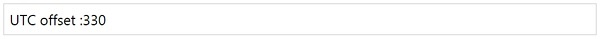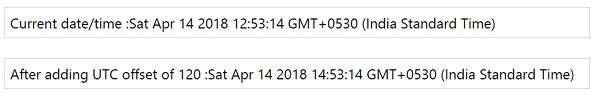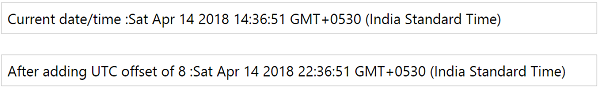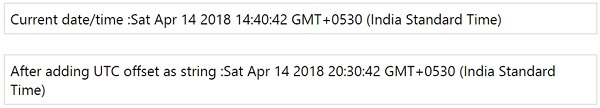# MomentJS - UTC offset

This method gives the display in minutes.

## Syntax

```moment().utcOffset();
moment().utcOffset(Number|String);
```

Observe the following examples for a better understanding −

## Example 1

```var day = moment().utcOffset();
```

## Output## Example 2

We can use pass value to utcOffset method as follows −

```var day = moment().utcOffset(120);
```

In the above, we are adding 120 minutes to the current moment and its output is displayed as below showing the current date/time and after adding offset.

## Output## Example 3

When the value given to the utcOffset is less than 16 and greater than -16, it will consider it as hours and the hours in the date are changed as shown below −

```var day = moment().utcOffset(8);
```

The output is as follows −

## OutputObserve that the current date shows hours as 14 and after adding 8 in utcOffset it changes the hours to 22.

## Example 4

We can also pass offset value as string as shown below −

```var day = moment().utcOffset("+05:50");
```

To the offset, we are adding 5 hrs and 50 mins as string and its is output as shown below −

## Outputmomentjs_manipulate_date_and_time.htm